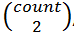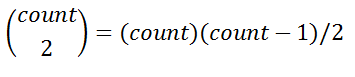Q:

# Count Substrings

Given a binary string find the number of substring that can formed such that every substring starts with "1" and ends with "1".

Example:

```    Input string:
100110100

Output:
6

Explanation:
1001
10011
1001101
11
1101
101
N.B: Don't think that substrings are:
1001
11
101
These only```

.

Algorithm:

Though it's a string problem, it can be solved actually using simple combinatorics.

1. Calculate total no of '1' occurring in the string
```Set count 0; (count is to store frequency of '1')
For each character at index i of the string
IF(string[i]=='1')
Increment count;
END IF
END FOR
```
1. Now we have total number of '1'. Finding no of substring starting with '1' & ending with '1' is equivalent of choosing two '1's out of total no of '1's (count). We are not at all bothered how many zeros are in between those two '1'. In fact, there can be '1's in between those two '1's picked up.
Thus,
number of substrings starting with '1' & ending with '1', where count is the frequency of '1's in the string.So for our above example:

```    Input string:
100110100
Number of '1's=4
Thus total number of substrings that can be formed
starting with '1' & ending with '1' = (4*3)/2 = 6
```

C++ implementation:

``````#include <bits/stdc++.h>
using namespace std;

int compute(string s){
int count=0;
//counting no of '1's
for(int i=0;i<s.length();i++)
if(s[i]=='1')
count++;
//returning count choose 2
return (count*(count-1))/2;
}

int main(){
string s;

cin>>s;

cout<<"no of substrings starting with '1' &";
cout<<" ending with '1' is: "<<compute(s)<<endl;

return 0;
}
``````

Output

```enter your input string(binary string)
100110100
no of substrings starting with '1' & ending with '1' is: 6```